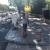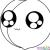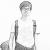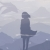# 几分钟内学习 Clojure已翻译 100%

oschina 投递于 2013/07/01 19:55 (共 2 段, 翻译完成于 07-02)

#### 参与翻译 (2人) : Sweelia, 凯奥斯

Clojure是JVM上的一个LISP语言变种。它比Common Lisp更强调纯函数式编程，但提供了一些STM工具以处理它所引入的状态问题。

（你需要Clojure 1.2或以上版本。

```; 分号作为注释的开始

; Clojure 用一种把元素用括号括起来的像列表一样的方式来书写，元素之间用空格隔开
; clojure 解释器会把第一个元素当做是函数或者宏调用，其他的都作为参数
; 下面这个函数用于设置当前的命名空间
(ns test)

; 更多基本的例子：

; str 函数会用它所有的参数创造一个字符串
(str "Hello" " " "World") ; => "Hello World"

; 数学运算很直观，不过是前缀表达式
(+ 1 1) ; => 2
(- 2 1) ; => 1
(* 1 2) ; => 2
(/ 2 1) ; => 2

;  相等比较使用 “=”符号
(= 1 1) ; => true
(= 2 1) ; => false

; 你也不必担心逻辑运算
(not true) ; => false

; 嵌套方式正如你预料的那样
(+ 1 (- 3 2)) ; = 1 + (3 - 2) => 2

; 类型系统
;;;;;;;;;;;;;

; Clojure 使用java对象类型来表示 布尔值、字符串和数字
; 使用 `class`函数来检测它们.
(class 1) ; 整形字面值默认是java中的Long类型
(class 1.); 浮点字面值对应着java中的Double类型
(class ""); 字符串总是用双引号括起来,并且对应着java中的Sring类型
(class false) ;布尔值对应着java中的Boolean类型
(class nil); null值被称为 nil（英语含义：无、零点）

; 如果你想创建一列数据字面值, 使用一个单引号 ' 来防表达式被解析执行
'(+ 1 2) ; => (+ 1 2) ;这里没有返回3
; (上面表达式和(quote (+ 1 2)) 等价，不过更简洁

; 你可以运算一个引用列表
(eval '(+ 1 2)) ; => 3

; 集合和序列
;;;;;;;;;;;;;;;;;;;

; 向量和列表也是java类哦！！
(class [1 2 3]); => clojure.lang.PersistentVector
(class '(1 2 3)); => clojure.lang.PersistentList

;书写一个列表形如(1 2 3)一样简单, 但是我们不得不把它“引”（前面加个单引号）起来
;这样就能防止解释器把它当做一个函数来解析
;另外，(list 1 2 3) 和 '(1 2 3) 等价

;列表和向量都是集合:
(coll? '(1 2 3)) ; => true
(coll? [1 2 3]) ; => true

; 只有列表是序列.（序列是有顺序的）
(seq? '(1 2 3)) ; => true
(seq? [1 2 3]) ; => false

; 序列是列表一种逻辑上的接口,可以懒加载.
; "懒" 意味着可以定义无穷序列,就像下面一样:
(range 4) ; => (0 1 2 3)
(range) ; => (0 1 2 3 4 ...) (一个无穷序列)
(take 4 (range)) ;  (0 1 2 3)

; 使用cons 来追加一个元素到列表或者向量的头部
(cons 4 [1 2 3]) ; => (4 1 2 3)
(cons 4 '(1 2 3)) ; => (4 1 2 3)

; 使用conj追加一个元素到列表的头部，或者向量的尾部,
(conj [1 2 3] 4) ; => [1 2 3 4]
(conj '(1 2 3) 4) ; => (4 1 2 3)

; 使用concat来连接列表和向量
(concat [1 2] '(3 4)) ; => (1 2 3 4)

; 使用filter, map 来进行列表计算
(map inc [1 2 3]) ; => (2 3 4)
(filter even? [1 2 3]) ; => (2)

; 使用reduce 来进行化繁为简  （map/reduce 思想就来自于lisp）
(reduce + [1 2 3 4])
; = (+ (+ (+ 1 2) 3) 4)
; => 10

; Reduce 可以使用一个初始值
(reduce conj [] '(3 2 1))
; = (conj (conj (conj [] 3) 2) 1)
; => [3 2 1]

; 函数
;;;;;;;;;;;;;;;;;;;;;

; 使用fn来创建一个函数。所有的函数都有返回值，就是它的最后一个表达式
(fn [] "Hello World") ; => fn

; (你需要额外的括号去调用它)
((fn [] "Hello World")) ; => "Hello World"

;你可以使用def来创建变量
(def x 1)
x ; => 1

; 将函数赋值给一个变量
(def hello-world (fn [] "Hello World"))
(hello-world) ; => "Hello World"

; 你可以使用defn来简化定义过程
(defn hello-world [] "Hello World")

;[] 是函数的参数列表
(defn hello [name]
(str "Hello " name))
(hello "Steve") ; => "Hello Steve"

; 你也可以使用下面这种简写方式
(def hello2 #(str "Hello " %1))
(hello2 "Fanny") ; => "Hello Fanny"

; 你可以创建拥有可变参数的函数
(defn hello3
([] "Hello World")
([name] (str "Hello " name)))
(hello3 "Jake") ; => "Hello Jake"
(hello3) ; => "Hello World"

; 函数允许将参数打包成列表 （有点类似python中的*）
(defn count-args [& args]
(str "You passed " (count args) " args: " args))
(count-args 1 2 3) ; => "You passed 3 args: (1 2 3)"

; 你可以将普通参数和列表参数混合使用
(defn hello-count [name & args]
(str "Hello " name ", you passed " (count args) " extra args"))
(hello-count "Finn" 1 2 3)
; => "Hello Finn, you passed 3 extra args"

; 哈希表
;;;;;;;;;;

(class {:a 1 :b 2 :c 3}) ; => clojure.lang.PersistentArrayMap

; 关键字类似字符串，但是做了一些性能上的优化
(class :a) ; => clojure.lang.Keyword

; Maps 的键可以是任意类型，但是通常推荐使用keywords
(def stringmap (hash-map "a" 1, "b" 2, "c" 3))
stringmap  ; => {"a" 1, "b" 2, "c" 3}

(def keymap (hash-map :a 1 :b 2 :c 3))
keymap ; => {:a 1, :c 3, :b 2} (不保证顺序)

; 顺便说一下, 逗号只是为了看着更清晰，其他都和空格一样，什么都不做.

; 从一个map中检索一个值，可以直接把这个map当做函数调用（这个NB）
(stringmap "a") ; => 1
(keymap :a) ; => 1

; 关键字也可以当做函数来调用，从一个map中检索值（这个更NB）
(:b keymap) ; => 2

; stings 可没有这个功能，所以下面会抛出异常。（这也是为什么推荐使用keywords）
;("a" stringmap)
; => Exception: java.lang.String cannot be cast to clojure.lang.IFn

; 检索一个不存在的值会返回nil
(stringmap "d") ; => nil

; 使用assoc 向一个map中添加新的键值对。
(assoc keymap :d 4) ; => {:a 1, :b 2, :c 3, :d 4}

; 请记住, clojure 类型是不可变的!
keymap ; => {:a 1, :b 2, :c 3}

; 使用dissoc 来删除key（可以删除多个）
(dissoc keymap :a :b) ; => {:c 3}

; 集合
;;;;;;

(class #{1 2 3}) ; => clojure.lang.PersistentHashSet
(set [1 2 3 1 2 3 3 2 1 3 2 1]) ; => #{1 2 3}

; 使用con来添加新值
(conj #{1 2 3} 4) ; => #{1 2 3 4}

; 使用disj删除原有值
(disj #{1 2 3} 1) ; => #{2 3}

; 直接将set当做函数来测试是否包含某个值（NB）
(#{1 2 3} 1) ; => 1  (有就返回原有的值）
(#{1 2 3} 4) ; => nil (没有就返回nil)

; clojure.sets 命名空间包含更多的函数

; 一些有用的形式
;;;;;;;;;;;;;;;;;

; clojure中的逻辑结构都是宏, 看起来也没什么不同
(if false "a" "b") ; => "b"
(if false "a") ; => nil

; 使用let 来创建临时绑定
(let [a 1 b 2]
(> a b)) ; => false

; 执行多条语句，返回最后一条语句
(do
(print "Hello")
"World") ; => "World" (prints "Hello")

; 所有的函数都包含一个隐式的do
(defn print-and-say-hello [name]
(print "Saying hello to " name)
(str "Hello " name))
(print-and-say-hello "Jeff") ;=> "Hello Jeff" (prints "Saying hello to Jeff")

; let绑定也是哦
(let [name "Urkel"]
(print "Saying hello to " name)
(str "Hello " name)) ; => "Hello Urkel" (prints "Saying hello to Urkel")

; 模块
;;;;;;;;;;;;;;;

; 使用“use”来获得一个模块中所有的函数
(use 'clojure.set)

; 现在我们可以使用集合操作
(intersection #{1 2 3} #{2 3 4}) ; => #{2 3}  求交集
(difference #{1 2 3} #{2 3 4}) ; => #{1}   求差集

; 你可以只导入一个函数子集（例如下面只包含交集函数）
(use '[clojure.set :only [intersection]])

; 使用reqire来导入一个模块
(require 'clojure.string)

; 使用/从一个模块中调用函数
(clojure.string/blank? "") ; => true

; 你可以在导入模块的时候自定义名称
(require '[clojure.string :as str])
(str/replace "This is a test." #"[a-o]" str/upper-case) ; => "THIs Is A tEst."
; (#"" denotes a regular expression literal)

; 你可以使用":require" 从一个命名空间中引入模块（use也可以，但是别这么做）
; 如果你使用:require的话，就没必要把模块“引”（前面加个单引号）起来了.
(ns test
(:require
[clojure.string :as str]
[clojure.set :as set]))

; Java
;;;;;;;;;;;;;;;;;

; java 拥有一个庞大的各种用途的标准库,你一定迫不及待想学习如何在clojure中使用这些库

; 使用import类引入java模块（这个还好没变化）
(import java.util.Date)

; 你也可以从一个命名空间中引入
(ns test
(:import java.util.Date
java.util.Calendar))

; 类名字后加个”."用来创建一个对象
(Date.) ; <a date object>

; 使用. 来调用方法. 或者使用“.方法名"简写的方式
(. (Date.) getTime) ; <a timestamp>
(.getTime (Date.)) ; 和上面一样哦

; 使用/ 来调用静态方法
(System/currentTimeMillis) ; <a timestamp> (system is always present)

; 使用 doto 来处理可变的类，所有的函数始终用最初的那个对象值，最后还是返回最初的那个对象  (import java.util.Calendar)
(doto (Calendar/getInstance)
(.set 2000 1 1 0 0 0)
.getTime) ; => A Date. set to 2000-01-01 00:00:00```

### 评论(63)

x

#### 引用来自“李旸”的评论#### 引用来自“lion_yang”的评论

C like =》1+2+3+4+5+6
list like =》(+ 1 2 3 4 5 6)s#### 引用来自“hantsy”的评论

+10000

+1 C++用熟了顶多也就是个高级码农，但是 Lisp研究透了就是个计算机科学家
+11+2*4/3+2+3+4+5
(+ 1 (/ (* 2 4) 3) 2 3 4 5)

1+2*4/3+2+3+(Math.pow(2,3))+Math.cos(5)
(+ 1 (/ (* 2 4) 3) 2 3 (pow 2 3) (cos 5))